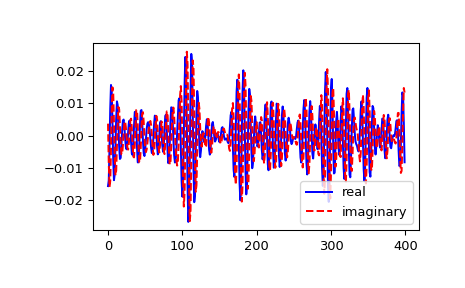# scipy.fft.ifft¶

scipy.fft.ifft(x, n=None, axis=- 1, norm=None, overwrite_x=False, workers=None, *, plan=None)[source]

Compute the 1-D inverse discrete Fourier Transform.

This function computes the inverse of the 1-D n-point discrete Fourier transform computed by fft. In other words, ifft(fft(x)) == x to within numerical accuracy.

The input should be ordered in the same way as is returned by fft, i.e.,

• x should contain the zero frequency term,

• x[1:n//2] should contain the positive-frequency terms,

• x[n//2 + 1:] should contain the negative-frequency terms, in increasing order starting from the most negative frequency.

For an even number of input points, x[n//2] represents the sum of the values at the positive and negative Nyquist frequencies, as the two are aliased together. See fft for details.

Parameters
xarray_like

Input array, can be complex.

nint, optional

Length of the transformed axis of the output. If n is smaller than the length of the input, the input is cropped. If it is larger, the input is padded with zeros. If n is not given, the length of the input along the axis specified by axis is used. See notes about padding issues.

axisint, optional

Axis over which to compute the inverse DFT. If not given, the last axis is used.

norm{“backward”, “ortho”, “forward”}, optional

Normalization mode (see fft). Default is “backward”.

overwrite_xbool, optional

If True, the contents of x can be destroyed; the default is False. See fft for more details.

workersint, optional

Maximum number of workers to use for parallel computation. If negative, the value wraps around from os.cpu_count(). See fft for more details.

plan: object, optional

This argument is reserved for passing in a precomputed plan provided by downstream FFT vendors. It is currently not used in SciPy.

New in version 1.5.0.

Returns
outcomplex ndarray

The truncated or zero-padded input, transformed along the axis indicated by axis, or the last one if axis is not specified.

Raises
IndexError

If axes is larger than the last axis of x.

fft

The 1-D (forward) FFT, of which ifft is the inverse.

ifft2

The 2-D inverse FFT.

ifftn

The N-D inverse FFT.

Notes

If the input parameter n is larger than the size of the input, the input is padded by appending zeros at the end. Even though this is the common approach, it might lead to surprising results. If a different padding is desired, it must be performed before calling ifft.

If x is a 1-D array, then the ifft is equivalent to

y[k] = np.sum(x * np.exp(2j * np.pi * k * np.arange(n)/n)) / len(x)


As with fft, ifft has support for all floating point types and is optimized for real input.

Examples

>>> import scipy.fft
>>> scipy.fft.ifft([0, 4, 0, 0])
array([ 1.+0.j,  0.+1.j, -1.+0.j,  0.-1.j]) # may vary


Create and plot a band-limited signal with random phases:

>>> import matplotlib.pyplot as plt
>>> t = np.arange(400)
>>> n = np.zeros((400,), dtype=complex)
>>> n[40:60] = np.exp(1j*np.random.uniform(0, 2*np.pi, (20,)))
>>> s = scipy.fft.ifft(n)
>>> plt.plot(t, s.real, 'b-', t, s.imag, 'r--')
[<matplotlib.lines.Line2D object at ...>, <matplotlib.lines.Line2D object at ...>]
>>> plt.legend(('real', 'imaginary'))
<matplotlib.legend.Legend object at ...>
>>> plt.show()scipy.fft.fft

scipy.fft.fft2